# Vector space

Vector space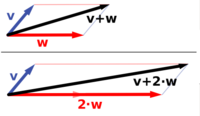Vector addition and scalar multiplication: a vector v (blue) is added to another vector w (red, upper illustration). Below, w is stretched by a factor of 2, yielding the sum v + 2·w.

A vector space is a mathematical structure formed by a collection of vectors: objects that may be added together and multiplied ("scaled") by numbers, called scalars in this context. Scalars are often taken to be real numbers, but one may also consider vector spaces with scalar multiplication by complex numbers, rational numbers, or even more general fields instead. The operations of vector addition and scalar multiplication have to satisfy certain requirements, called axioms, listed below. An example of a vector space is that of Euclidean vectors which are often used to represent physical quantities such as forces: any two forces (of the same type) can be added to yield a third, and the multiplication of a force vector by a real factor is another force vector. In the same vein, but in more geometric parlance, vectors representing displacements in the plane or in three-dimensional space also form vector spaces.

Vector spaces are the subject of linear algebra and are well understood from this point of view, since vector spaces are characterized by their dimension, which, roughly speaking, specifies the number of independent directions in the space. The theory is further enhanced by introducing on a vector space some additional structure, such as a norm or inner product. Such spaces arise naturally in mathematical analysis, mainly in the guise of infinite-dimensional function spaces whose vectors are functions. Analytical problems call for the ability to decide if a sequence of vectors converges to a given vector. This is accomplished by considering vector spaces with additional data, mostly spaces endowed with a suitable topology, thus allowing the consideration of proximity and continuity issues. These topological vector spaces, in particular Banach spaces and Hilbert spaces, have a richer theory.

Historically, the first ideas leading to vector spaces can be traced back as far as 17th century's analytic geometry, matrices, systems of linear equations, and Euclidean vectors. The modern, more abstract treatment, first formulated by Giuseppe Peano in the late 19th century, encompasses more general objects than Euclidean space, but much of the theory can be seen as an extension of classical geometric ideas like lines, planes and their higher-dimensional analogs.

Today, vector spaces are applied throughout mathematics, science and engineering. They are the appropriate linear-algebraic notion to deal with systems of linear equations; offer a framework for Fourier expansion, which is employed in image compression routines; or provide an environment that can be used for solution techniques for partial differential equations. Furthermore, vector spaces furnish an abstract, coordinate-free way of dealing with geometrical and physical objects such as tensors. This in turn allows the examination of local properties of manifolds by linearization techniques. Vector spaces may be generalized in several directions, leading to more advanced notions in geometry and abstract algebra.

## Introduction and definition

### First example: arrows in the plane

The concept of vector space will first be explained by describing two particular examples. The first example of a vector space consists of arrows in a fixed plane, starting at one fixed point. This is used in physics to describe forces or velocities. Given any two such arrows, v and w, the parallelogram spanned by these two arrows contains one diagonal arrow that starts at the origin, too. This new arrow is called the sum of the two arrows and is denoted v + w. Another operation that can be done with arrows is scaling: given any positive real number a, the arrow that has the same direction as v, but is dilated or shrunk by multiplying its length by a, is called multiplication of v by a. It is denoted a · v. When a is negative, a · v is defined as the arrow pointing in the opposite direction, instead.

The following shows a few examples: if a = 2, the resulting vector a · w has the same direction as w, but is stretched to the double length of w (right image below). Equivalently 2 · w is the sum w + w. Moreover, (−1) · v = −v has the opposite direction and the same length as v (blue vector pointing down in the right image).

### Second example: ordered pairs of numbers

A second key example of a vector space is provided by pairs of real numbers x and y. (The order of the components x and y is significant, so such a pair is also called an ordered pair.) Such a pair is written as (x, y). The sum of two such pairs and multiplication of a pair with a number is defined as follows:

(x1, y1) + (x2, y2) = (x1 + x2, y1 + y2)

and

a · (x, y) = (ax, ay).

### Definition

A vector space over a field F is a set V together with two binary operators that satisfy the eight axioms listed below. Elements of V are called vectors. Elements of F are called scalars. In this article, vectors are differentiated from scalars by boldface.[nb 1] In the two examples above, our set consists of the planar arrows with fixed starting point and of pairs of real numbers, respectively, while our field is the real numbers. The first operation, vector addition, takes any two vectors v and w and assigns to them a third vector which is commonly written as v + w, and called the sum of these two vectors. The second operation takes any scalar a and any vector v and gives another vector a · v. In view of the first example, where the multiplication is done by rescaling the vector v by a scalar a, the multiplication is called scalar multiplication of v by a.

To qualify as a vector space, the set V and the operations of addition and multiplication have to adhere to a number of requirements called axioms. In the list below, let u, v, w be arbitrary vectors in V, and a, b be scalars in F.

 Axiom Signification Associativity of addition v1 + (v2 + v3) = (v1 + v2) + v3. Commutativity of addition v1 + v2 = v2 + v1. Identity element of addition There exists an element 0 ∈ V, called the zero vector, such that v + 0 = v for all v ∈ V. Inverse elements of addition For all v ∈ V, there exists an element -v ∈ V, called the additive inverse of v, such that v + (-v) = 0. Distributivity of scalar multiplication with respect to vector addition s(v1 + v2) = sv1 + sv2. Distributivity of scalar multiplication with respect to field addition (n1 + n2)v = n1v + n2v. Respect of scalar multiplication over field's multiplication n1 (n2 s) = (n1 n2)s [nb 2] Identity element of scalar multiplication 1s = s, where 1 denotes the multiplicative identity in F.

These axioms generalize properties of the vectors introduced in the above examples. Indeed, the result of addition of two ordered pairs (as in the second example above) does not depend on the order of the summands:

(xv, yv) + (xw, yw) = (xw, yw) + (xv, yv),

Likewise, in the geometric example of vectors as arrows, v + w = w + v, since the parallelogram defining the sum of the vectors is independent of the order of the vectors. All other axioms can be checked in a similar manner in both examples. Thus, by disregarding the concrete nature of the particular type of vectors, the definition incorporates these two and many more examples in one notion of vector space.

Subtraction of two vectors and division by a (non-zero) scalar can be performed via

vw = v + (−w),
v / a = (1 / a) · v.

The concept introduced above is called a real vector space. The word "real" refers to the fact that vectors can be multiplied by real numbers, as opposed to, say, complex numbers. When scalar multiplication is defined for complex numbers, the denomination complex vector space is used. These two cases are the ones used most often in engineering. The most general definition of a vector space allows scalars to be elements of a fixed field F. Then, the notion is known as F-vector spaces or vector spaces over F. A field is, essentially, a set of numbers possessing addition, subtraction, multiplication and division operations.[nb 3] For example, rational numbers also form a field.

In contrast to the intuition stemming from vectors in the plane and higher-dimensional cases, there is, in general vector spaces, no notion of nearness, angles or distances. To deal with such matters, particular types of vector spaces are introduced; see below.

### Alternative formulations and elementary consequences

The requirement that vector addition and scalar multiplication be binary operations includes (by definition of binary operations) a property called closure: that u + v and av are in V for all a in F, and u, v in V. Some older sources mention these properties as separate axioms.

In the parlance of abstract algebra, the first four axioms can be subsumed by requiring the set of vectors to be an abelian group under addition. The remaining axioms give this group an F-module structure. In other words there is a ring homomorphism ƒ from the field F into the endomorphism ring of the group of vectors. Then scalar multiplication av is defined as (ƒ(a))(v).

There are a number of direct consequences of the vector space axioms. Some of them derive from elementary group theory, applied to the additive group of vectors: for example the zero vector 0 of V and the additive inverse −v of any vector v are unique. Other properties follow from the distributive law, for example av equals 0 if and only if a equals 0 or v equals 0.

## History

Vector spaces stem from affine geometry, via the introduction of coordinates in the plane or three-dimensional space. Around 1636, Descartes and Fermat founded analytic geometry by equating solutions to an equation of two variables with points on a plane curve. To achieve geometric solutions without using coordinates, Bolzano introduced, in 1804, certain operations on points, lines and planes, which are predecessors of vectors. This work was made use of in the conception of barycentric coordinates by Möbius in 1827. The foundation of the definition of vectors was Bellavitis' notion of the bipoint, an oriented segment one of whose ends is the origin and the other one a target. Vectors were reconsidered with the presentation of complex numbers by Argand and Hamilton and the inception of quaternions and biquaternions by the latter. They are elements in R2, R4, and R8; treating them using linear combinations goes back to Laguerre in 1867, who also defined systems of linear equations.

In 1857, Cayley introduced the matrix notation which allows for a harmonization and simplification of linear maps. Around the same time, Grassmann studied the barycentric calculus initiated by Möbius. He envisaged sets of abstract objects endowed with operations. In his work, the concepts of linear independence and dimension, as well as scalar products are present. Actually Grassmann's 1844 work exceeds the framework of vector spaces, since his considering multiplication, too, led him to what are today called algebras. Peano was the first to give the modern definition of vector spaces and linear maps in 1888.

An important development of vector spaces is due to the construction of function spaces by Lebesgue. This was later formalized by Banach and Hilbert, around 1920. At that time, algebra and the new field of functional analysis began to interact, notably with key concepts such as spaces of p-integrable functions and Hilbert spaces. Vector spaces, including infinite-dimensional ones, then became a firmly established notion, and many mathematical branches started making use of this concept.

## Examples

### Coordinate and function spaces

The first example of a vector space over a field F is the field itself, equipped with its standard addition and multiplication. This is the case n = 1 of a vector space usually denoted Fn, known as the coordinate space whose elements are n-tuples (sequences of length n):

(a1, a2, ..., an), where the ai are elements of F.

The case F = R and n = 2 was discussed in the introduction above. Infinite coordinate sequences, and more generally functions from any fixed set Ω to a field F also form vector spaces, by performing addition and scalar multiplication pointwise. That is, the sum of two functions ƒ and g is given by

(ƒ + g)(w) = ƒ(w) + g(w)

and similarly for multiplication. Such function spaces occur in many geometric situations, when Ω is the real line or an interval, or other subsets of Rn. Many notions in topology and analysis, such as continuity, integrability or differentiability are well-behaved with respect to linearity: sums and scalar multiples of functions possessing such a property still have that property. Therefore, the set of such functions are vector spaces. They are studied in greater detail using the methods of functional analysis, see below. Algebraic constraints also yield vector spaces: the vector space F[x] is given by polynomial functions:

ƒ(x) = r0 + r1x + ... + rn−1xn−1 + rnxn, where the coefficients r0, ..., rn are in F.

### Linear equations

Systems of homogeneous linear equations are closely tied to vector spaces. For example, the solutions of

 a + 3b + c = 0 4a + 2b + 2c = 0

are given by triples with arbitrary a, b = a/2, and c = −5a/2. They form a vector space: sums and scalar multiples of such triples still satisfy the same ratios of the three variables; thus they are solutions, too. Matrices can be used to condense multiple linear equations as above into one vector equation, namely

Ax = 0,

where A =$\begin{bmatrix} 1 & 3 & 1 \\ 4 & 2 & 2\end{bmatrix}$ is the matrix containing the coefficients of the given equations, x is the vector (a, b, c), Ax denotes the matrix product and 0 = (0, 0) is the zero vector. In a similar vein, the solutions of homogeneous linear differential equations form vector spaces. For example

ƒ''(x) + 2ƒ'(x) + ƒ(x) = 0

yields ƒ(x) = a ex + bx ex, where a and b are arbitrary constants, and ex is the natural exponential function.

### Field extensions

Field extensions F / E ("F over E") provide another class of examples of vector spaces, particularly in algebra and algebraic number theory: a field F containing a smaller field E becomes an E-vector space, by the given multiplication and addition operations of F. For example the complex numbers are a vector space over R. A particularly interesting type of field extension in number theory is Q(α), the extension of the rational numbers Q by a fixed complex number α. Q(α) is the smallest field containing the rationals and a fixed complex number α. Its dimension as a vector space over Q depends on the choice of α.

## Bases and dimension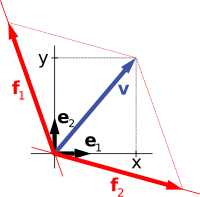A vector v in R2 (blue) expressed in terms of different bases: using the standard basis of R2 v = xe1 + ye2 (black), and using a different, non-orthogonal basis: v = f1 + f2 (red).

Bases reveal the structure of vector spaces in a concise way. A basis is defined as a (finite or infinite) set B = {vi}iI of vectors vi indexed by some index set I that spans the whole space, and is minimal with this property. The former means that any vector v can be expressed as a finite sum (called linear combination) of the basis elements$v = a_1 \mathbf{v}_{i_1} + a_2 \mathbf{v}_{i_2} + \cdots + a_n \mathbf{v}_{i_n}$,

where the ak are scalars and vik (k = 1, ..., n) elements of the basis B. Minimality, on the other hand, is made formal by requiring B to be linearly independent. A set of vectors is said to be linearly independent if none of its elements can be expressed as a linear combination of the remaining ones. Equivalently, an equation$a_1 \mathbf{v}_{i_1} + a_2 \mathbf{v}_{i_2} + \cdots + a_n \mathbf{v}_{i_n} = 0$

can only hold if all scalars a1, ..., an equal zero. Linear independence ensures that the representation of any vector in terms of basis vectors, the existence of which is guaranteed by the requirement that the basis span V, is unique. This is referred to as the coordinatized viewpoint of vector spaces, by viewing basis vectors as generalizations of coordinate vectors x, y, z in R3 and similarly in higher-dimensional cases.

The coordinate vectors e1 = (1, 0, ..., 0), e2 = (0, 1, 0, ..., 0), to en = (0, 0, ..., 0, 1), form a basis of Fn, called the standard basis, since any vector (x1, x2, ..., xn) can be uniquely expressed as a linear combination of these vectors:

(x1, x2, ..., xn) = x1(1, 0, ..., 0) + x2(0, 1, 0, ..., 0) + ... + xn(0, ..., 0, 1) = x1e1 + x2e2 + ... + xnen.

Every vector space has a basis. This follows from Zorn's lemma, an equivalent formulation of the axiom of choice. Given the other axioms of Zermelo-Fraenkel set theory, the existence of bases is equivalent to the axiom of choice. The ultrafilter lemma, which is weaker than the axiom of choice, implies that all bases of a given vector space have the same number of elements, or cardinality. It is called the dimension of the vector space, denoted dim V. If the space is spanned by finitely many vectors, the above statements can be proven without such fundamental input from set theory.

The dimension of the coordinate space Fn is n, by the basis exhibited above. The dimension of the polynomial ring F[x] introduced above is countably infinite, a basis is given by 1, x, x2, ... A fortiori, the dimension of more general function spaces, such as the space of functions on some (bounded or unbounded) interval, is infinite.[nb 4] Under suitable regularity assumptions on the coefficients involved, the dimension of the solution space of a homogeneous ordinary differential equation equals the degree of the equation. For example, the solution space for the above equation is generated by ex and xex. These two functions are linearly independent over R, so the dimension of this space is two, as is the degree of the equation.

The dimension (or degree) of the field extension Q(α) over Q depends on α. If α satisfies some polynomial equation

qnαn + qn−1αn−1 + ... + q0 = 0, with rational coefficients qn, ..., q0.

("α is algebraic"), the dimension is finite. More precisely, it equals the degree of the minimal polynomial having α as a root. For example, the complex numbers C are a two-dimensional real vector space, generated by 1 and the imaginary unit i. The latter satisfies i2 + 1 = 0, an equation of degree two. Thus, C is a two-dimensional R-vector space (and, as any field, one-dimensional as a vector space over itself, C). If α is not algebraic, the dimension of Q(α) over Q is infinite. For instance, for α = π there is no such equation, in other words π is transcendental.

## Linear maps and matrices

The relation of two vector spaces can be expressed by linear map or linear transformation. They are functions that reflect the vector space structure—i.e., they preserve sums and scalar multiplication:

ƒ(x + y) = ƒ(x) + ƒ(y) and ƒ(a · x) = a · ƒ(x) for all x and y in V, all a in F.

An isomorphism is a linear map ƒ : VW such that there exists an inverse map g : WV, which is a map such that the two possible compositions ƒg : WW and gƒ : VV are identity maps. Equivalently, ƒ is both one-to-one (injective) and onto (surjective). If there exists an isomorphism between V and W, the two spaces are said to be isomorphic; they are then essentially identical as vector spaces, since all identities holding in V are, via ƒ, transported to similar ones in W, and vice versa via g.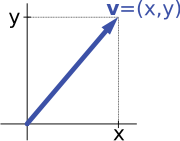Describing an arrow vector v by its coordinates x and y yields an isomorphism of vector spaces.

For example, the vector spaces in the introduction are isomorphic: a planar arrow v departing at the origin of some (fixed) coordinate system can be expressed as an ordered pair by considering the x- and y-component of the arrow, as shown in the image at the right. Conversely, given a pair (x, y), the arrow going by x to the right (or to the left, if x is negative), and y up (down, if y is negative) turns back the arrow v.

Linear maps VW between two fixed vector spaces form a vector space HomF(V, W), also denoted L(V, W). The space of linear maps from V to F is called the dual vector space, denoted V. Via the injective natural map VV∗∗, any vector space can be embedded into its bidual; the map is an isomorphism if and only if the space is finite-dimensional.

Once a basis of V is chosen, linear maps ƒ : VW are completely determined by specifying the images of the basis vectors, because any element of V is expressed uniquely as a linear combination of them. If dim V = dim W, a 1-to-1 correspondence between fixed bases of V and W gives rise to a linear map that maps any basis element of V to the corresponding basis element of W. It is an isomorphism, by its very definition. Therefore, two vector spaces are isomorphic if their dimensions agree and vice versa. Another way to express this is that any vector space is completely classified (up to isomorphism) by its dimension, a single number. In particular, any n-dimensional F-vector space V is isomorphic to Fn. There is, however, no "canonical" or preferred isomorphism; actually an isomorphism φ: FnV is equivalent to the choice of a basis of V, by mapping the standard basis of Fn to V, via φ. Appending an automorphism, i.e. an isomorphism ψ: VV yields another isomorphism ψ∘φ: FnV, the composition of ψ and φ, and therefore a different basis of V.[citation needed] The freedom of choosing a convenient basis is particularly useful in the infinite-dimensional context, see below.

### Matrices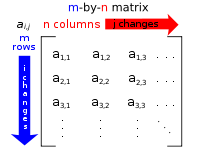A typical matrix

Matrices are a useful notion to encode linear maps. They are written as a rectangular array of scalars as in the image at the right. Any m-by-n matrix A gives rise to a linear map from Fn to Fm, by the following$\mathbf x = (x_1, x_2, \cdots, x_n) \mapsto \left(\sum_{j=1}^n a_{1j}x_j, \sum_{j=1}^n a_{2j}x_j, \cdots, \sum_{j=1}^n a_{mj}x_j \right)$, where$\sum$ denotes summation,

or, using the matrix multiplication of the matrix A with the coordinate vector x:

xAx.

Moreover, after choosing bases of V and W, any linear map ƒ : VW is uniquely represented by a matrix via this assignment.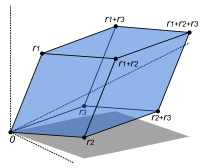The volume of this parallelepiped is the absolute value of the determinant of the 3-by-3 matrix formed by the vectors r1, r2, and r3.

The determinant det (A) of a square matrix A is a scalar that tells whether the associated map is an isomorphism or not: to be so it is sufficient and necessary that the determinant is nonzero. The linear transformation of Rn corresponding to a real n-by-n matrix is orientation preserving if and only if the determinant is positive.[citation needed]

### Eigenvalues and eigenvectors

Endomorphisms, linear maps ƒ : VV, are particularly important since in this case vectors v can be compared with their image under ƒ, ƒ(v). Any nonzero vector v satisfying λv = ƒ(v), where λ is a scalar, is called an eigenvector of ƒ with eigenvalue λ.[nb 5] Equivalently, v is an element of the kernel of the difference ƒλ · Id (where Id is the identity map VV). If V is finite-dimensional, this can be rephrased using determinants: ƒ having eigenvalue λ is equivalent to

det (ƒλ · Id) = 0.

By spelling out the definition of the determinant, the expression on the left hand side can be seen to be a polynomial function in λ, called the characteristic polynomial of ƒ. If the field F is large enough to contain a zero of this polynomial (which automatically happens for F algebraically closed, such as F = C) any linear map has at least one eigenvector. The vector space V may or may not possess an eigenbasis, a basis consisting of eigenvectors. This phenomenon is governed by the Jordan canonical form of the map.[nb 6] The set of all eigenvectors corresponding to a particular eigenvalue of ƒ forms a vector space known as the eigenspace corresponding to the eigenvalue (and ƒ) in question. To achieve the spectral theorem, the corresponding statement in the infinite-dimensional case, the machinery of functional analysis is needed, see below.

## Basic constructions

In addition to the above concrete examples, there are a number of standard linear algebraic constructions that yield vector spaces related to given ones. In addition to the definitions given below, they are also characterized by universal properties, which determine an object X by specifying the linear maps from X to any other vector space.

### Subspaces and quotient spaces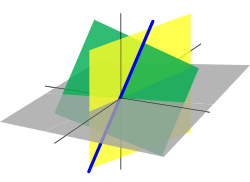A line passing through the origin (blue, thick) in R3 is a linear subspace. It is the intersection of two planes (green and yellow).

A nonempty subset W of a vector space V that is closed under addition and scalar multiplication (and therefore contains the 0-vector of V) is called a subspace of V. Subspaces of V are vector spaces (over the same field) in their own right. The intersection of all subspaces containing a given set S of vectors is called its span, and is the smallest subspace of V containing the set S. Expressed in terms of elements, the span is the subspace consisting of all the linear combinations of elements of S.

The counterpart to subspaces are quotient vector spaces. Given any subspace WV, the quotient space V/W ("V modulo W") is defined as follows: as a set, it consists of v + W = {v + w, wW}, where v is an arbitrary vector in V. The sum of two such elements v1 + W and v2 + W is (v1 + v2) + W, and scalar multiplication is given by a · (v + W) = (a · v) + W. The key point in this definition is that v1 + W = v2 + W if and only if the difference of v1 and v2 lies in W.[nb 7] This way, the quotient space "forgets" information that is contained in the subspace W.

The kernel ker(ƒ) of a linear map ƒ: VW consists of vectors v that are mapped to 0 in W. Both kernel and image im(ƒ) = {ƒ(v), vV} are subspaces of V and W, respectively. The existence of kernels and images is part of the statement that the category of vector spaces (over a fixed field F) is an abelian category, i.e. a corpus of mathematical objects and structure-preserving maps between them (a category) that behaves much like the category of abelian groups. Because of this, many statements such as the first isomorphism theorem (also called rank-nullity theorem in matrix-related terms)

V / ker(ƒ) ≅ im(ƒ).

and the second and third isomorphism theorem can be formulated and proven in a way very similar to the corresponding statements for groups.

An important example is the kernel of a linear map xAx for some fixed matrix A, as above. The kernel of this map is the subspace of vectors x such that Ax = 0, which is precisely the set of solutions to the system of homogeneous linear equations belonging to A. This concept also extends to linear differential equations$a_0 f + a_1 \frac{d f}{d x} + a_2 \frac{d^2 f}{d x^2} + \cdots + a_n \frac{d^n f}{d x^n} = 0$, where the coefficients ai are functions in x, too.

In the corresponding map$f \mapsto D(f) = \sum_{i=0}^n a_i \frac{d^i f}{d x^i}$,

the derivatives of the function ƒ appear linearly (as opposed to ƒ''(x)2, for example). Since differentiation is a linear procedure (i.e., (ƒ + g)' = ƒ' + g ' and (c·ƒ)' = c·ƒ' for a constant c) this assignment is linear, called a linear differential operator. In particular, the solutions to the differential equation D(ƒ) = 0 form a vector space (over R or C).

### Direct product and direct sum

The direct product$\textstyle{\prod_{i \in I} V_i}$ of a family of vector spaces Vi consists of the set of all tuples (vi)iI, which specify for each index i in some index set I an element vi of Vi. Addition and scalar multiplication is performed componentwise. A variant of this construction is the direct sum$\oplus_{i \in I} V_i$ (also called coproduct and denoted$\textstyle{\coprod_{i \in I}V_i}$), where only tuples with finitely many nonzero vectors are allowed. If the index set I is finite, the two constructions agree, but differ otherwise.

### Tensor product

The tensor product VF W, or simply VW, of two vector spaces V and W is one of the central notions of multilinear algebra which deals with extending notions such as linear maps to several variables. A map g: V × WX is called bilinear if g is linear in both variables v and w. That is to say, for fixed w the map vg(v, w) is linear in the sense above and likewise for fixed v.

The tensor product is a particular vector space that is a universal recipient of bilinear maps g, as follows. It is defined as the vector space consisting of finite (formal) sums of symbols called tensors

v1w1 + v2w2 + ... + vnwn,

subject to the rules

a · (vw) = (a · v) ⊗ w = v ⊗ (a · w), where a is a scalar,
(v1 + v2) ⊗ w = v1w + v2w, and
v ⊗ (w1 + w2) = vw1 + vw2.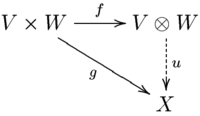Commutative diagram depicting the universal property of the tensor product.

These rules ensure that the map ƒ from the V × W to VW that maps a tuple (v, w) to vw is bilinear. The universality states that given any vector space X and any bilinear map g: V × WX, there exists a unique map u, shown in the diagram with a dotted arrow, whose composition with ƒ equals g: u(vw) = g(v, w). This is called the universal property of the tensor product, an instance of the method—much used in advanced abstract algebra—to indirectly define objects by specifying maps from or to this object.

## Vector spaces with additional structure

From the point of view of linear algebra, vector spaces are completely understood insofar as any vector space is characterized, up to isomorphism, by its dimension. However, vector spaces ad hoc do not offer a framework to deal with the question—crucial to analysis—whether a sequence of functions converges to another function. Likewise, linear algebra is not adapted to deal with infinite series, since the addition operation allows only finitely many terms to be added. Therefore, the needs of functional analysis require considering additional structures. Much the same way the axiomatic treatment of vector spaces reveals their essential algebraic features, studying vector spaces with additional data abstractly turns out to be advantageous, too.[citation needed]

A first example of an additional datum is an order ≤, a token by which vectors can be compared. For example, n-dimensional real space Rn can be ordered by comparing its vectors componentwise. Ordered vector spaces, for example Riesz spaces, are fundamental to Lebesgue integration, which relies on the ability to express a function as a difference of two positive functions

ƒ = ƒ+ƒ,

where ƒ+ denotes the positive part of ƒ and ƒ the negative part.

### Normed vector spaces and inner product spaces

"Measuring" vectors is done by specifying a norm, a datum which measures lengths of vectors, or by an inner product, which measures angles between vectors. Norms and inner products are denoted$| \mathbf v|$ and$\lang \mathbf v | \mathbf w \rang$, respectively. The datum of an inner product entails that lengths of vectors can be defined too, by defining the associated norm$|\mathbf v| := \sqrt {\langle \mathbf v | \mathbf v \rangle}$. Vector spaces endowed with such data are known as normed vector spaces and inner product spaces, respectively.

Coordinate space Fn can be equipped with the standard dot product:$\lang \mathbf x | \mathbf y \rang = \mathbf x \cdot \mathbf y = x_1 y_1 + \cdots + x_n y_n.$

In R2, this reflects the common notion of the angle between two vectors x and y, by the law of cosines:$\mathbf x \cdot \mathbf y = \cos\left(\angle (\mathbf x, \mathbf y)\right) \cdot |\mathbf x| \cdot |\mathbf y|.$

Because of this, two vectors satisfying$\lang \mathbf x | \mathbf y \rang = 0$ are called orthogonal. An important variant of the standard dot product is used in Minkowski space: R4 endowed with the Lorentz product$\lang \mathbf x | \mathbf y \rang = x_1 y_1 + x_2 y_2 + x_3 y_3 - x_4 y_4.$

In contrast to the standard dot product, it is not positive definite:$\lang \mathbf x | \mathbf x \rang$ also takes negative values, for example for x = (0, 0, 0, 1). Singling out the fourth coordinate—corresponding to time, as opposed to three space-dimensions—makes it useful for the mathematical treatment of special relativity.

### Topological vector spaces

Convergence questions are treated by considering vector spaces V carrying a compatible topology, a structure that allows one to talk about elements being close to each other. Compatible here means that addition and scalar multiplication have to be continuous maps. Roughly, if x and y in V, and a in F vary by a bounded amount, then so do x + y and ax.[nb 8] To make sense of specifying the amount a scalar changes, the field F also has to carry a topology in this context; a common choice are the reals or the complex numbers.

In such topological vector spaces one can consider series of vectors. The infinite sum$\sum_{i=0}^{\infty} f_i$

denotes the limit of the corresponding finite partial sums of the sequence (ƒi)iN of elements of V. For example, the ƒi could be (real or complex) functions belonging to some function space V, in which case the series is a function series. The mode of convergence of the series depends on the topology imposed on the function space. In such cases, pointwise convergence and uniform convergence are two prominent examples.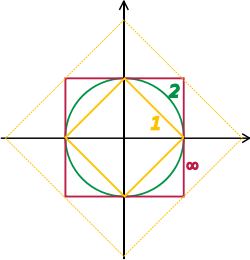Unit "spheres" in R2 consist of plane vectors of norm 1. Depicted are the unit spheres in different p-norms, for p = 1, 2, and ∞. The bigger diamond depicts points of 1-norm equal to$\sqrt 2$.

A way to ensure the existence of limits of certain infinite series is to restrict attention to spaces where any Cauchy sequence has a limit; such a vector space is called complete. Roughly, a vector space is complete provided that it contains all necessary limits. For example, the vector space of polynomials on the unit interval [0,1], equipped with the topology of uniform convergence is not complete because any continuous function on [0,1] can be uniformly approximated by a sequence of polynomials, by the Weierstrass approximation theorem. In contrast, the space of all continuous functions on [0,1] with the same topology is complete. A norm gives rise to a topology by defining that a sequence of vectors vn converges to v if and only if$\text{lim}_{n \rightarrow \infty} |\mathbf v_n - \mathbf v| = 0.$

Banach and Hilbert spaces are complete topological spaces whose topologies are given, respectively, by a norm and an inner product. Their study—a key piece of functional analysis—focusses on infinite-dimensional vector spaces, since all norms on finite-dimensional topological vector spaces give rise to the same notion of convergence. The image at the right shows the equivalence of the 1-norm and ∞-norm on R2: as the unit "balls" enclose each other, a sequence converges to zero in one norm if and only if it so does in the other norm. In the infinite-dimensional case, however, there will generally be inequivalent topologies, which makes the study of topological vector spaces richer than that of vector spaces without additional data.

From a conceptual point of view, all notions related to topological vector spaces should match the topology. For example, instead of considering all linear maps (also called functionals) VW, maps between topological vector spaces are required to be continuous. In particular, the (topological) dual space V consists of continuous functionals VR (or C). The fundamental Hahn–Banach theorem is concerned with separating subspaces of appropriate topological vector spaces by continuous functionals.

#### Banach spaces

Banach spaces, introduced by Stefan Banach, are complete normed vector spaces. A first example is the vector space ℓ p consisting of infinite vectors with real entries x = (x1, x2, ...) whose p-norm (1 ≤ p ≤ ∞) given by$|\mathbf x|_p := \left(\sum_i |x_i|^p \right)^{1/p}$ for p < ∞ and$|\mathbf x|_\infty := \text{sup}_i |x_i|$

is finite. The topologies on the infinite-dimensional space ℓ p are inequivalent for different p. E.g. the sequence of vectors xn = (2n, 2n, ..., 2n, 0, 0, ...), i.e. the first 2n components are 2n, the following ones are 0, converges to the zero vector for p = ∞, but does not for p = 1:$|x_n|_\infty = \sup (2^{-n}, 0) = 2^{-n} \rightarrow 0$, but$|x_n|_1 = \sum_{i=1}^{2^n} 2^{-n} = 2^n \cdot 2^{-n} = 1.$

More generally than sequences of real numbers, functions ƒ: Ω → R are endowed with a norm that replaces the above sum by the Lebesgue integral$|f|_p := \left(\int_\Omega |f(x)|^p \, dx \right)^{1/p}.$

The space of integrable functions on a given domain Ω (for example an interval) satisfying |ƒ|p < ∞, and equipped with this norm are called Lebesgue spaces, denoted Lp(Ω).[nb 9] These spaces are complete. (If one uses the Riemann integral instead, the space is not complete, which may be seen as a justification for Lebesgue's integration theory.[nb 10]) Concretely this means that for any sequence of Lebesgue-integrable functions ƒ1, ƒ2, ... with |ƒn|p < ∞, satisfying the condition$\lim_{k,\ n \to \infty}\int_\Omega |{f}_k (x)-{f}_n (x)|^p \, dx = 0$

there exists a function ƒ(x) belonging to the vector space Lp(Ω) such that$\lim_{k \to \infty}\int_\Omega |{f} (x)-{f}_k (x)|^p \, dx = 0.\$

Imposing boundedness conditions not only on the function, but also on its derivatives leads to Sobolev spaces.

#### Hilbert spaces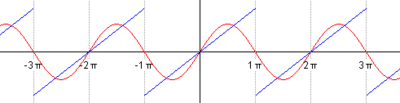The succeeding snapshots show summation of 1 to 5 terms in approximating a periodic function (blue) by finite sum of sine functions (red).

Complete inner product spaces are known as Hilbert spaces, in honor of David Hilbert. The Hilbert space L2(Ω), with inner product given by$\langle f\ , \ g \rangle = \int_\Omega f(x) \overline{g(x)} \, dx,\,\!$

where$\overline{g(x)}$ denotes the complex conjugate of g(x).[nb 11] is a key case.

By definition, in a Hilbert space any Cauchy sequences converges to a limit. Conversely, finding a sequence of functions ƒn with desirable properties that approximates a given limit function, is equally crucial. Early analysis, in the guise of the Taylor approximation, established an approximation of differentiable functions ƒ by polynomials. By the Stone–Weierstrass theorem, every continuous function on [a, b] can be approximated as closely as desired by a polynomial. A similar approximation technique by trigonometric functions is commonly called Fourier expansion, and is much applied in engineering, see below. More generally, and more conceptually, the theorem yields a simple description of what "basic functions", or, in abstract Hilbert spaces, what basic vectors suffice to generate a Hilbert space H, in the sense that the closure of their span (i.e., finite linear combinations and limits of those) is the whole space. Such a set of functions is called a basis of H, its cardinality is known as the Hilbert dimension.[nb 12] Not only does the theorem exhibit suitable basis functions as sufficient for approximation purposes, together with the Gram-Schmidt process it also allows to construct a basis of orthogonal vectors. Such orthogonal bases are the Hilbert space generalization of the coordinate axes in finite-dimensional Euclidean space.

The solutions to various differential equations can be interpreted in terms of Hilbert spaces. For example, a great many fields in physics and engineering lead to such equations and frequently solutions with particular physical properties are used as basis functions, often orthogonal. As an example from physics, the time-dependent Schrödinger equation in quantum mechanics describes the change of physical properties in time, by means of a partial differential equation whose solutions are called wavefunctions. Definite values for physical properties such as energy, or momentum, correspond to eigenvalues of a certain (linear) differential operator and the associated wavefunctions are called eigenstates. The spectral theorem decomposes a linear compact operator acting on functions in terms of these eigenfunctions and their eigenvalues.

### Algebras over fields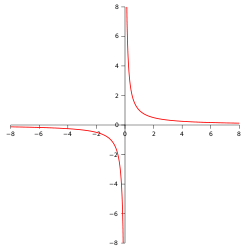A hyperbola, given by the equation x · y = 1. The coordinate ring of functions on this hyperbola is given by R[x, y] / (x · y − 1), an infinite-dimensional vector space over R.

General vector spaces do not possess a multiplication operation. A vector space equipped with an additional bilinear operator defining the multiplication of two vectors is an algebra over a field. Many algebras stem from functions on some geometrical object: since functions with values in a field can be multiplied, these entities form algebras. The Stone–Weierstrass theorem mentioned above, for example, relies on Banach algebras which are both Banach spaces and algebras.

Commutative algebra makes great use of rings of polynomials in one or several variables, introduced above. Their multiplication is both commutative and associative. These rings and their quotients form the basis of algebraic geometry, because they are rings of functions of algebraic geometric objects.

Another crucial example are Lie algebras, which are neither commutative nor associative, but the failure to be so is limited by the constraints ([x, y] denotes the product of x and y):

Examples include the vector space of n-by-n matrices, with [x, y] = xyyx, the commutator of two matrices, and R3, endowed with the cross product.

The tensor algebra T(V) is a formal way of adding products to any vector space V to obtain an algebra. As a vector space, it is spanned by symbols, called simple tensors

v1v2 ⊗ ... ⊗ vn, where the degree n varies.

The multiplication is given by concatenating such symbols, imposing the distributive law under addition, and requiring that scalar multiplication commute with the tensor product ⊗, much the same way as with the tensor product of two vector spaces introduced above. In general, there are no relations between v1v2 and v2v1. Forcing two such elements to be equal leads to the symmetric algebra, whereas forcing v1v2 = − v2v1 yields the exterior algebra.

When a field, F is explicitly stated, a common term used is F-algebra.

## Applications

Vector spaces have manifold applications as they occur in many circumstances, namely wherever functions with values in some field are involved. They provide a framework to deal with analytical and geometrical problems, or are used in the Fourier transform. This list is not exhaustive: many more applications exist, for example in optimization. The minimax theorem of game theory stating the existence of a unique payoff when all players play optimally can be formulated and proven using vector spaces methods. Representation theory fruitfully transfers the good understanding of linear algebra and vector spaces to other mathematical domains such as group theory.

### Distributions

A distribution (or generalized function) is a linear map assigning a number to each "test" function, typically a smooth function with compact support, in a continuous way: in the above terminology the space of distributions is the (continuous) dual of the test function space. The latter space is endowed with a topology that takes into account not only ƒ itself, but also all its higher derivatives. A standard example is the result of integrating a test function ƒ over some domain Ω:$I(f) = \int_\Omega f(x)\,dx.$

When Ω = {p}, the set consisting of a single point, this reduces to the Dirac distribution, denoted by δ, which associates to a test function ƒ its value at the p: δ(ƒ) = ƒ(p). Distributions are a powerful instrument to solve differential equations. Since all standard analytic notions such as derivatives are linear, they extend naturally to the space of distributions. Therefore the equation in question can be transferred to a distribution space, which is bigger than the underlying function space, so that more flexible methods are available for solving the equation. For example, Green's functions and fundamental solutions are usually distributions rather than proper functions, and can then be used to find solutions of the equation with prescribed boundary conditions. The found solution can then in some cases be proven to be actually a true function, and a solution to the original equation (e.g., using the Lax–Milgram theorem, a consequence of the Riesz representation theorem).

### Fourier analysisThe heat equation describes the dissipation of physical properties over time, such as the decline of the temperature of a hot body placed in a colder environment (yellow depicts colder regions than red).

Resolving a periodic function into a sum of trigonometric functions forms a Fourier series, a technique much used in physics and engineering.[nb 13] The underlying vector space is usually the Hilbert space L2(0, 2π), for which the functions sin mx and cos mx (m an integer) form an orthogonal basis. The Fourier expansion of an L2 function f is$\frac{a_0}{2} + \sum_{m=1}^{\infty}\left[a_m\cos\left(mx\right)+b_m\sin\left(mx\right)\right].$

The coefficients am and bm are called Fourier coefficients of ƒ, and are calculated by the formulas$a_m = \frac{1}{\pi} \int_0^{2 \pi} f(t) \cos (mt) \, dt$,$b_m = \frac{1}{\pi} \int_0^{2 \pi} f(t) \sin (mt) \, dt.$

In physical terms the function is represented as a superposition of sine waves and the coefficients give information about the function's frequency spectrum. A complex-number form of Fourier series is also commonly used. The concrete formulae above are consequences of a more general mathematical duality called Pontryagin duality. Applied to the group R, it yields the classical Fourier transform; an application in physics are reciprocal lattices, where the underlying group is a finite-dimensional real vector space endowed with the additional datum of a lattice encoding positions of atoms in crystals.

Fourier series are used to solve boundary value problems in partial differential equations. In 1822, Fourier first used this technique to solve the heat equation. A discrete version of the Fourier series can be used in sampling applications where the function value is known only at a finite number of equally spaced points. In this case the Fourier series is finite and its value is equal to the sampled values at all points. The set of coefficients is known as the discrete Fourier transform (DFT) of the given sample sequence. The DFT is one of the key tools of digital signal processing, a field whose applications include radar, speech encoding, image compression. The JPEG image format is an application of the closely related discrete cosine transform.

The fast Fourier transform is an algorithm for rapidly computing the discrete Fourier transform. It is used not only for calculating the Fourier coefficients but, using the convolution theorem, also for computing the convolution of two finite sequences. They in turn are applied in digital filters and as a rapid multiplication algorithm for polynomials and large integers (Schönhage-Strassen algorithm).

### Differential geometry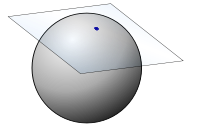The tangent space to the 2-sphere at some point is the infinite plane touching the sphere in this point.

The tangent plane to a surface at a point is naturally a vector space whose origin is identified with the point of contact. The tangent plane is the best linear approximation, or linearization, of a surface at a point.[nb 14] Even in a three-dimensional Euclidean space, there is typically no natural way to prescribe a basis of the tangent plane, and so it is conceived of as an abstract vector space rather than a real coordinate space. The tangent space is the generalization to higher-dimensional differentiable manifolds.

Riemannian manifolds are manifolds whose tangent spaces are endowed with a suitable inner product. Derived therefrom, the Riemann curvature tensor encodes all curvatures of a manifold in one object, which finds applications in general relativity, for example, where the Einstein curvature tensor describes the matter and energy content of space-time. The tangent space of a Lie group can be given naturally the structure of a Lie algebra and can be used to classify compact Lie groups.

## Generalizations

### Vector bundles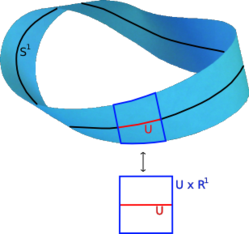A Möbius strip. Locally, it looks like U × R.

A vector bundle is a family of vector spaces parametrized continuously by a topological space X. More precisely, a vector bundle over X is a topological space E equipped with a continuous map

π : EX

such that for every x in X, the fiber π−1(x) is a vector space. The case dim V = 1 is called a line bundle. For any vector space V, the projection X × VX makes the product X × V into a "trivial" vector bundle. Vector bundles over X are required to be locally a product of X and some (fixed) vector space V: for every x in X, there is a neighborhood U of x such that the restriction of π to π−1(U) is isomorphic[nb 15] to the trivial bundle U × VU. Despite their locally trivial character, vector bundles may (depending on the shape of the underlying space X) be "twisted" in the large, i.e., the bundle need not be (globally isomorphic to) the trivial bundle X × V. For example, the Möbius strip can be seen as a line bundle over the circle S1 (by identifying open intervals with the real line). It is, however, different from the cylinder S1 × R, because the latter is orientable whereas the former is not.

Properties of certain vector bundles provide information about the underlying topological space. For example, the tangent bundle consists of the collection of tangent spaces parametrized by the points of a differentiable manifold. The tangent bundle of the circle S1 is globally isomorphic to S1 × R, since there is a global nonzero vector field on S1.[nb 16] In contrast, by the hairy ball theorem, there is no (tangent) vector field on the 2-sphere S2 which is everywhere nonzero. K-theory studies the isomorphism classes of all vector bundles over some topological space. In addition to deepening topological and geometrical insight, it has purely algebraic consequences, such as the classification of finite-dimensional real division algebras: R, C, the quaternions H and the octonions.[citation needed]

The cotangent bundle of a differentiable manifold consists, at every point of the manifold, of the dual of the tangent space, the cotangent space. Sections of that bundle are known as differential one-forms.

### Modules

Modules are to rings what vector spaces are to fields. The very same axioms, applied to a ring R instead of a field F yield modules. The theory of modules, compared to vector spaces, is complicated by the presence of ring elements that do not have multiplicative inverses. For example, modules need not have bases, as the Z-module (i.e., abelian group) Z/2Z shows; those modules that do (including all vector spaces) are known as free modules.

To see why the requirement that for every scalar there exist multiplicative inverse makes a difference, consider this: the fact that every vector space has a basis amounts to saying that every vector space is a free module. The proof of this fact fails for general modules over any ring(at least for modules of finite type) in the step "in every list b of linearly dependent vectors, it is possible to remove one vector from b without changing span of b". This does not hold in a general module, but holds in any vector space. So when we have a linearly dependent finite list of vectors that spans the whole vector space at hand (which must exist in a finite-dimensional vector space), we can remove one vector from the list and the list will still span the whole vector space. We can repeat this process until we get a basis; we cannot do this in general in a module. As it turns out, requiring that a module be over a field instead of a ring is a sufficient condition for this to hold.

Nevertheless, a vector space can be compactly defined as a module over a ring which is a field with the elements being called vectors. The algebro-geometric interpretation of commutative rings via their spectrum allows the development of concepts such as locally free modules, the algebraic counterpart to vector bundles.

### Affine and projective spaces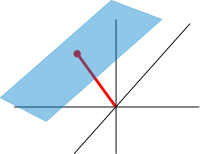An affine plane (light blue) in R3. It is a two-dimensional subspace shifted by a vector x (red).

Roughly, affine spaces are vector spaces whose origin is not specified. More precisely, an affine space is a set with a free transitive vector space action. In particular, a vector space is an affine space over itself, by the map

V × VV, (v, a) ↦ a + v.

If W is a vector space, then an affine subspace is a subset of W obtained by translating a linear subspace V by a fixed vector xW; this space is denoted by x + V (it is a coset of V in W) and consists of all vectors of the form x + v for vV. An important example is the space of solutions of a system of inhomogeneous linear equations

Ax = b

generalizing the homogeneous case b = 0 above. The space of solutions is the affine subspace x + V where x is a particular solution of the equation, and V is the space of solutions of the homogeneous equation (the nullspace of A).

The set of one-dimensional subspaces of a fixed finite-dimensional vector space V is known as projective space; it may be used to formalize the idea of parallel lines intersecting at infinity. Grassmannians and flag manifolds generalize this by parametrizing linear subspaces of fixed dimension k and flags of subspaces, respectively.

### Convex analysis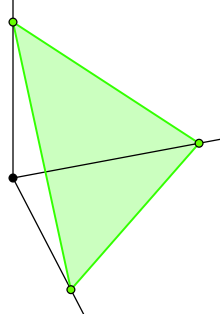The n-simplex is the standard convex set, that maps to every polytope, and is the intersection of the standard (n + 1) affine hyperplane (standard affine space) and the standard (n + 1) orthant (standard cone).

Over an ordered field, notably the real numbers, there are the added notions of convex analysis, most basically a cone, which allows only non-negative linear combinations, and a convex set, which allows only non-negative linear combinations that sum to 1. A convex set can be seen as the combinations of the axioms for an affine space and a cone, which is reflected in the standard space for it, the n-simplex, being the intersection of the affine hyperplane and orthant. Such spaces are particularly used in linear programming.

In the language of universal algebra, a vector space is an algebra over the universal vector space K of finite sequences of coefficients, corresponding to finite sums of vectors, while an affine space is an algebra over the universal affine hyperplane in here (of finite sequences summing to 1), a cone is an algebra over the universal orthant, and a convex set is an algebra over the universal simplex. This geometrizes the axioms in terms of "sums with (possible) restrictions on the coordinates".

Many concepts in linear algebra have analogs in convex analysis, including basic ones such as basis and span (such as in the form of convex hull), and notably including duality (in the form of dual polyhedron, dual cone, dual problem). Unlike linear algebra, however, where every vector space or affine space is isomorphic to the standard spaces, not every convex set or cone is isomorphic to the simplex or orthant. Rather, there is always a map from the simplex onto a polytope, given by generalized barycentric coordinates, and a dual map from a polytope into the orthant (of dimension equal to the number of faces) given by slack variables, but these are rarely isomorphisms – most polytopes are not a simplex or an orthant.

 Cartesian coordinate system Euclidean vector, for vectors in physics Gyrovector space

Wikimedia Foundation. 2010.

### Look at other dictionaries:

• vector space — Math. an additive group in which addition is commutative and with which is associated a field of scalars, as the field of real numbers, such that the product of a scalar and an element of the group or a vector is defined, the product of two… …   Universalium

• vector space — noun a) A type of set of vectors that satisfies a specific group of constraints. A vector space is a set of vectors which can be linearly combined. b) A set V, whose elements are called vectors , together with a binary operation + forming a… …   Wiktionary

• vector space — vektorių erdvė statusas T sritis radioelektronika atitikmenys: angl. vector space vok. Vektorraum, m rus. векторное пространство, n; пространство векторов, n pranc. espace vectoriel, m …   Radioelektronikos terminų žodynas

• vector space — vektorinė erdvė statusas T sritis fizika atitikmenys: angl. vector space vok. Vektorraum, m rus. векторное пространство, n pranc. espace vectoriel, m …   Fizikos terminų žodynas

• Vector space model — (or term vector model ) is an algebraic model for representing text documents (and any objects, in general) as vectors of identifiers, such as, for example, index terms. It is used in information filtering, information retrieval, indexing and… …   Wikipedia

• vector space — noun Date: 1937 a set of vectors along with operations of addition and multiplication such that the set is a commutative group under addition, it includes a multiplicative inverse, and multiplication by scalars is both associative and… …   New Collegiate Dictionary

• vector space — noun : a set representing a generalization of a system of vectors and consisting of elements which comprise a commutative group under addition, each of which is left unchanged under multiplication by the multiplicative identity of a field, and… …   Useful english dictionary

• Topological vector space — In mathematics, a topological vector space is one of the basic structures investigated in functional analysis. As the name suggests the space blends a topological structure (a uniform structure to be precise) with the algebraic concept of a… …   Wikipedia

• Normed vector space — In mathematics, with 2 or 3 dimensional vectors with real valued entries, the idea of the length of a vector is intuitive and can easily be extended to any real vector space Rn. The following properties of vector length are crucial. 1. The zero… …   Wikipedia

• Locally convex topological vector space — In functional analysis and related areas of mathematics, locally convex topological vector spaces or locally convex spaces are examples of topological vector spaces (TVS) which generalize normed spaces. They can be defined as topological vector… …   Wikipedia